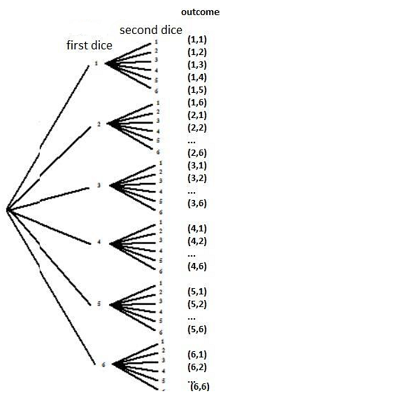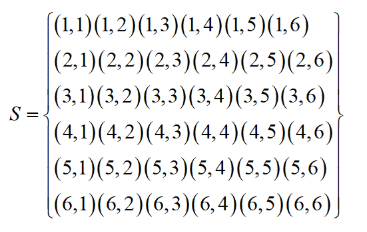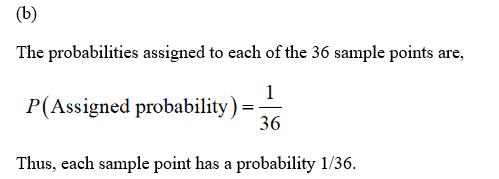# Two fair dice are tossed, and the face on each die is observed.Use a tree diagram to find the 36 sample points contained in the sample space.Assign probabilities to the sample points in part a.Find the probability of each of the following events:A = {3 showing on each die}B = {sum of two numbers showing is 7}C = {sum of two numbers showing is even}Also, for events A, B, and C, which one is simple event, which one is compoundevent?Event D= ? , Event D and event C independent or not? Event D and event Bmutually exclusive or not?Consider event D and event C, Find the probability of each of the followingevents: ?(D ∩ ?), p(D ∪ ?), ?(?|?), ?(?/|?/), ?(?/ ∩ ?/)

Question
16 views
1. Two fair dice are tossed, and the face on each die is observed.

1. Use a tree diagram to find the 36 sample points contained in the sample space.

2. Assign probabilities to the sample points in part a.

3. Find the probability of each of the following events:

A = {3 showing on each die}
B = {sum of two numbers showing is 7}
C = {sum of two numbers showing is even}

4. Also, for events A, B, and C, which one is simple event, which one is compound

event?

5. Event D= ? , Event D and event C independent or not? Event D and event B

mutually exclusive or not?

6. Consider event D and event C, Find the probability of each of the following

events: ?(D ∩ ?), p(D ∪ ?), ?(?|?), ?(?/|?/), ?(?/ ∩ ?/)

check_circle

Step 1

"Since you have posted a question with multiple sub-parts, we will solve first three sub-parts for you. To get remaining sub-part solved please repost the complete question and mention the sub-parts to be solved."

Step 2

(a)

The tree diagram showing the sample points are given below:The sample space containing the 36 sample points when rolling two dices are,Thus, the 36 sample points are stated.

Step 3...

### Want to see the full answer?

See Solution

#### Want to see this answer and more?

Solutions are written by subject experts who are available 24/7. Questions are typically answered within 1 hour.*

See Solution
*Response times may vary by subject and question.
Tagged in